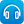# Mathematics / Year 7 / Number and Algebra / Number and place value

Version 8
Curriculum content descriptions

Investigate index notation and represent whole numbers as products of powers of prime numbers (ACMNA149)

Elaborations
• defining and comparing prime and composite numbers and explaining the difference between them
• applying knowledge of factors to strategies for expressing whole numbers as products of powers of prime factors, such as repeated division by prime factors or creating factor trees
• solving problems involving lowest common multiples and greatest common divisors (highest common factors) for pairs of whole numbers by comparing their prime factorisation
General capabilities
• Numeracy Numeracy
ScOT terms

Indices,  Prime numbers

## Refine by topic

Related topic### Numbers Count: What are factors?

What are factors? Watch as the jelly babies in this clip show you! What are the factors of 12? How many factors does the number 11 have? Try explaining to a friend what a prime number is.### Catalyst: Small scale measurements

What units of measurements do we use to describe incredibly small things like blood cells and atoms? Watch as you are taken on a journey to explain the different units of measurement that we use to describe the very small.### Catalyst: Prime numbers and unbreakable codes

Imagine if anyone was able to read all our secret, encrypted messages and information. Watch and find out how scientists at the Australian National University are developing a new encryption system using quantum physics and quantum computing.### Self Improvement Wednesday: The beauty of prime numbers

A prime number is a number that only has two factors: one and itself. Listen to Adam Spencer and Richard Glover discussing prime numbers. They cover how we define these numbers and how and why prime numbers are widely used in internet encryption.### MathXplosion, Ep 6: Zero the hero

What is the role of zero as a placeholder for large numbers such as 1 million, 1 billion and 1 trillion? Find out about the notion of place value and powers of ten through the act of bead counting.### Patterns, primes and Pascal's Triangle

Are you intrigued by patterns? Check out Vi Hart as she explains how to visualise patterns in prime numbers, using Ulam's Spiral. Watch as Vi creates patterns, using Pascal's Triangle to explore relationships in number. See what happens when she circles the odd numbers. What rule does she use to create the final pattern?### MathXplosion, Ep 7: The power of exponents

Have you heard of the term "exponential growth"? Growth can occur very quickly when powers are involved. See how you can use the power of two to rapidly increase the amount of anything from grain to coins!### reSolve: Prime factorisation

This sequence of four lessons explores prime factorisation. Students solve a puzzle using factor strings, play a dice game to learn about prime numbers, develop a method for finding all of the factors of a number, and engage in an investigation of highest common factors and lowest common multiples of two numbers, and how ...### Catalyst: Graham's number

If you were asked what the biggest number you can think of is, what would you say? Infinity? Well, what about the biggest finite number you can think of? Mathematician Ron Graham came across such a gigantic number in his research that, to capture its massive size, he and his colleagues needed to come up with new methods ...### Understanding exponents: 2

This resource is a short video presentation, with audio commentary, in which the meaning of exponents or powers of a number is explained. In the numerical example used the presenter explains the difference between evaluating the power of a number and the product of two numbers.### Secondary mathematics: using real data

These seven learning activities, which focus on the use of 'real data' using a variety of tools (software) and devices (hardware), illustrate the ways in which content, pedagogy and technology can be successfully and effectively integrated in order to promote learning. In the activities, teachers use the three content strands ...### reSolve: Real numbers - Sum of squares

This lesson explores Diophantus' hypothesis that all natural numbers can be expressed as the sum of no more than four square numbers. Students are invited to work in small groups to express natural numbers up to 120 as a sum of squares and share this on a whole-class grid. The resource develops students' fluency in identifying ...### Square roots and square numbers

This is a website designed for both teachers and students that deals with square roots of square numbers from the Australian Curriculum for year 7 students. It contains material on products of squares and square roots, prime factorisation and square roots of non-perfect squares. There are pages for both teachers and students. ...### The laws of arithmetic and their use in algebra

This is a website designed for both teachers and students that refers to algebraic notation, the laws of arithmetic and the use of these laws in algebra from the Australian Curriculum for year 7 students. It contains material on algebraic notation, the commutative and associative laws, the use of brackets and the orders ...